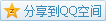# MIT OCW

## 18.02 Multivariable Calculus

### Dot Product

#### Definition

For two $n$-dimensional vectors $\mathbf{a,b}$, their dot product is defined as $$\mathbf{a}\cdot\mathbf{b}=\sum a_ib_i$$ and is equivalent to the following definition:
$$\mathbf{a}\cdot\mathbf{b}=|\mathbf{a}||\mathbf{b}|\cos\theta$$ where $\theta$ is the angle between $\mathbf{a},\mathbf{b}$. (Definition 2 is more realistic in a plane or space.)

#### Application

• Angles
Derived from the definition 2, it's easy to get the cosine of the angle like $$\cos\theta=\frac{\mathbf{a}\cdot\mathbf{b}}{|\mathbf{a}||\mathbf{b}|}.$$ Thus given two concrete vectors in space, the angle between them can be easily found after their dot product is calculated by definition 1 and measured by Pythagoras.
• Orthogonality Detection
If $\mathbf{a}\cdot\mathbf{b}=0$, then $\cos\theta=0$, which implies that $\theta=\frac\pi2$, namely, $\mathbf{a}\perp\mathbf{b}$.
Actually the sign of $\mathbf{a}\cdot\mathbf{b}$ is the same as $\cos\theta$ since $|a||b|$ has to be positive.
e.g. What does the solution set of $x+2y+3z=0$ in space look like?
Select a point $P(x,y,z)$ and the equation means that $\mathbf{OP}\cdot(1,2,3)=0$, which also means that $\mathbf{OP}\perp(1,2,3)$ and thus it's much more visual that the content is a plane.
• Extract Components
For a vector $\mathbf{a}$ and a unit vector $\hat\mathbf{e}$, the component on $\hat\mathbf{e}$'s direction is just $\mathbf{a}\cdot\hat\mathbf{e}$.

• Area
For a triangle composed of vectors $\mathbf{a},\mathbf{b}$, its area should be $S=\frac12|\mathbf{a}||\mathbf{b}|\sin\theta$. Anyhow $\sin\theta$ is actually not that easy to get. So we try to convert $\sin\theta$ to $\cos\theta'$ where $\sin\theta=\cos\theta'$, it's easy to construct a $\theta'=\theta-\frac\pi2$.
Thus $S=\frac12|\mathbf{a}||\mathbf{b}|\cos\theta'$, meanwhile, we need to find some $\mathbf{a'}$ whose length is just $|\mathbf{a}|$ and the angle between $\mathbf{a'}$ and $\mathbf{b}$ should be $\theta'$.
In Cartesian plane, a vector $\mathbf{x}=(x_1,x_2)$ becomes $\mathbf{x'}=(-x_2,x_1)$ after rotated counterclockwise.
Therefore, if $\mathbf{a}=(a_1,a_2)$ and $\mathbf{b}=(b_1,b_2)$, we can find the substitute vector $\mathbf{a'}=(-a_2,a_1)$ and the area of the triangle is $S=\frac12|\mathbf{a'}\cdot\mathbf{b}|=\frac12|a_1b_2-a_2b_1|$. (The absolute value ensures that the area won't become negative.)
For some polygon, we can just divide the polygon into some triangles and repeatedly use the method to get the whole area.

### Determinant (2D, 3D)

#### Definition

For vectors $\mathbf{a}=(a_1,a_2),\mathbf{b}=(b_1,b_2)$, the determinant $$\det(\mathbf{a},\mathbf{b})=\begin{vmatrix}a_1 & a_2\\b_1 & b_2\end{vmatrix}=a_1b_2-a_2b_1.$$
Having such symbolic notation, we can write the area expression above as $$S=\frac12\begin{Vmatrix}a_1 & a_2 \\ b_1 & b_2\end{Vmatrix}=\frac12|\det(\mathbf{a},\mathbf{b})|.$$
For a vector $\mathbf{a}=(a_1,a_2,a_3),\mathbf{b}=(b_1,b_2,b_3),\mathbf{c}=(c_1,c_2,c_3)$, the determinant $$\det(\mathbf{a},\mathbf{b},\mathbf{c})=\begin{vmatrix}a_1 & a_2 & a_3 \\ b_1 & b_2 & b_3 \\ c_1 & c_2 & c_3\end{vmatrix}=a_1\begin{vmatrix}b_2 & b_3 \\ c_2 & c_3\end{vmatrix}-a_2\begin{vmatrix}b_1 & b_3 \\ c_1 & c_3\end{vmatrix}+a_3\begin{vmatrix}b_1 & b_2 \\ c_1 & c_2\end{vmatrix}.$$

#### Geometry

The determinant $\det(\mathbf{a},\mathbf{b},\mathbf{c})$ is just the volume of parallelepiped boxed by $\mathbf{a},\mathbf{b},\mathbf{c}$.

#### Application

• Plane Equation
Suppose there are three different points $A,B,C$ (determining a plane exactly), and we want to know the equation of that plane. Select a point $P(x,y,z)$ on the plane then the parallelepiped boxed by $\mathbf{AP},\mathbf{AB},\mathbf{AC}$ should have no volume, which generates the equation that is $\det(\mathbf{AP},\mathbf{AB},\mathbf{AC})=0$, you can simplify it as long as you'd love.

### Cross Product

#### Definition

Given two vectors $\mathbf{a}=(a_1,a_2,a_3),\mathbf{b}=(b_1,b_2,b_3)$, their cross product $\mathbf{a}\times\mathbf{b}=\begin{vmatrix} \hat\mathbf{i} & \hat\mathbf{j} & \hat\mathbf{k} \\ a_1 & a_2 & a_3 \\ b_1 & b_2 & b_3 \end{vmatrix}$.

#### Geometry

If the area of the parallelogram composed of $\mathbf{a},\mathbf{b}$ is $S$, then $|\mathbf{a}\times\mathbf{b}|=S$. In terms of the direction, it holds that $\mathbf{a}\times\mathbf{b}\perp\mathbf{a}$ and $\mathbf{a}\times\mathbf{b}\perp\mathbf{b}$, namely, the cross product is perpendicular to the plane which $\mathbf{a},\mathbf{b}$ determine.
Concretely, the actual direction has two possible results, which can be determined uniquely through Right-hand Rule.
Memorization Given a special case that is the $\hat\mathbf{i}\times\hat\mathbf{j}=\hat\mathbf{k}$.

#### Application

• Volume
For a parallelepiped boxed by $\mathbf{a},\mathbf{b},\mathbf{c}$, its volume is exactly the base area times the height, more importantly, the base is actually a parallelogram. Without loss of generality, suppose the base is $\mathbf{b}$ and $\mathbf{c}$, thus the base area is $|\mathbf{b}\times\mathbf{c}|$.
However, the height should be converted to a component on some "vertical" direction, namely which is perpendicular to the base.
We can use the cross product again and change it into some unit vector to get the direction that is $$\hat\mathbf{n}=\frac{\mathbf{b}\times\mathbf{c}}{|\mathbf{b}\times\mathbf{c}|}.$$
Therefore the height is $\mathbf{a}\cdot\hat\mathbf{n}$.
Finally, the volume can be expressed as $$V=|\mathbf{b}\times\mathbf{c}|\left(\mathbf{a}\cdot\frac{\mathbf{b}\times\mathbf{c}}{|\mathbf{b}\times\mathbf{c}|}\right)=\mathbf{a}\cdot(\mathbf{b}\times\mathbf{c}).$$ and it's equivalent to $\det(\mathbf{a},\mathbf{b},\mathbf{c})$

• Plane Equation
The same prerequisites as above, and $P$ is still on the plane. Given the vector $\mathbf{n}$ which is perpendicular to the plane, it holds that $\mathbf{AP}\cdot\mathbf{n}=0$ since $\mathbf{n}$ should be perpendicular to any vector on the plane, where$\mathbf{n}=\mathbf{AB}\times\mathbf{AC}$.